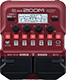# Speech

Discussion in 'Zoom B1/B1X Four' started by Erwin Zettlmeier, Dec 26, 2020.

1. SpeechDevice: Zoom B1 Four
Firmware: 2.00

Name on device: Speech
Optimized for: Phones/Speaker

Effects chain:Speech

Effect: "LMT-76" (Dynamics), active - "yes"
"Input" = 74
"Ratio" = 8:1
"REL" = 10
"Output" = 31

Effect: "Z-Syn" (Sfx), active - "yes"
"FREQ" = 6
"Range" = 11
"Decay" = 76
"RESO" = 12
"Wave" = 0
"Tone" = 5
"BAL" = 62
"VOL" = 56
"Frequency" = 0
"Range" = 0
"Decay" = 0
"Resonance" = 0
"Wave" = SAW
"Tone" = 0
"Balance" = 0
"Volume" = 0

Effect: "Phaser" (Modulation), active - "yes"
"Color" = INV 8
"Depth" = 100
"Rate" = 12
"Resonance" = 50

Effect: "PitchSHFT" (Modulation), active - "yes"
"Shift" = 12
"Fine" = 0
"Tone" = 3
"Balance" = 14

Effect: "HD Hall" (Reverb), active - "yes"
"PreD" = 94
"Decay" = 0
"Mix" = 52
"Tail" = On

Patch Volume: 44